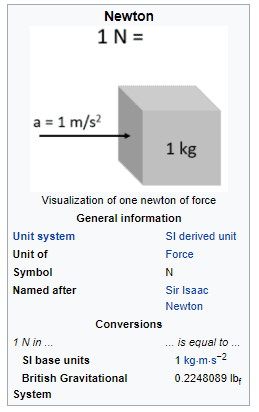# Moment of Inertia and units

• RundleSeb

#### RundleSeb

TL;DR Summary
Calculating moment of inertia and translating it between units
Calculating moment of inertia and translating it between units, I've become confused.

The example is a mass of 1kg at 2000mm from the pivot. The force is applied at 1000mm from the pivot.

Basics as far as I'm aware:
Moment of inertia = mass * Distance to center of rotation^2
Torque = Moment of inertia * angular acceleration.
Torque = Force* The distance of the force to the center of rotation

so to achieve acceleration of 1 rad/sec^2

In meters
Moment of inertia = 1*(2^2) = 4 kg m^2
Torque = Moment of inertia * angular acceleration = 4*1 = 4 Nm
Force = 4 NM / 1m = 4N

In mm

Moment of inertia = 1*(2000^2) = 4000,000 kg mm^2
Torque = Moment of inertia * angular acceleration = 4000,000*1 = 4000,000 Nmm
Force = 4000,000 NM /1000 mm = 4000N

Can the calculation not be done in distance units other than meters? am I missing something?

Torque units are wrong. 1 kg mm2/s2 ≠ 1 N mm.

I assume as such, but I can't find equations other than those.

But you can do the units conversion, can't you? That's all that's wrong.

Hmm

Force = mass*acceleration = mass*(distance/time^2)
Force * distance = mass*distance^2 * 1/time^2

It seems the units do kinda add up? I think?

After a bit of thought over the units, I currently believe this discrepancy is because

1 N = 1 (kg*m)/s^2
thus 1 N = 1000 (kg*mm)/s^2 (or, 1 (kg*mm)/s^2 = 1μN)

Thus considering the earlier question,
Torque (Nmm) = Moment of inertia (kg*mm^2) * angular acceleration(1/s^2) = 4000,000*1 = 4000,000 (μN mm) or 4000 (N mm)

Force = mass*acceleration = mass*(distance/time^2)
Force * distance = mass*distance^2 * 1/time^2

It seems the units do kinda add up? I think?
That's dimensions, not units. A picometre and a light year both have dimensions of length, but have very different magnitudes.
Your last post is not quite right. (It might just be a typo)
4,000,000 kg mm2 * 1 s-2 = 4,000,000 kg mm s-2*mm = 4,000,000 mN mm = 4000 N mm = 4 N m.

Ah i get what you were going at now.

Also well spotted (it is a typo , should be mN not μN)

The inertia definition is integral of I= r^2dm and the units of this is [Kg*sqrm]
the inertia of rectangle is : I= B*H^3/12 and the unit of inertia is [m^4]

My question is how do the two things work out?
Is there no contradiction between them?

The inertia definition is integral of I= r^2dm and the units of this is [Kg*sqrm]
the inertia of rectangle is : I= B*H^3/12 and the unit of inertia is [m^4]

My question is how do the two things work out?
Is there no contradiction between them?